# Steenrod reduced power

A stable cohomology operation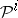,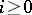, of the type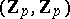, whereis a fixed odd prime number, which is the analogue moduloof the Steenrod square, and which is a homomorphism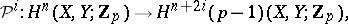defined for every pair of topological spaces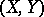and any integer. The Steenrod reduced powers possess the following properties (apart from naturality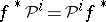and stability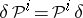, where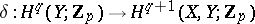is the coboundary homomorphism):

1)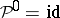;

2) if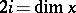, then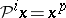;

3) if, then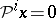;

4) (Cartan's formula)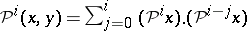;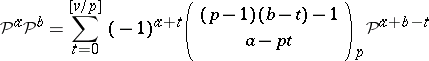if,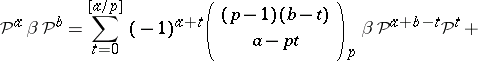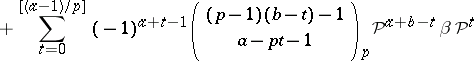if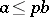, whereis the Bockstein homomorphism associated with the short exact sequence of coefficient groups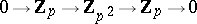, while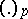are the binomial coefficients reduced modulo.

These properties are analogous to the corresponding properties of Steenrod squares, whereby the operationcorresponds to the operation. Just as for Steenrod squares, the multiplication in 4) can be considered to be both exterior (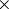-multiplication) and interior (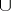-multiplication). Steenrod reduced powers commute with suspension and transgression.

The properties 1)–3) uniquely characterize, and can be constructed in the same way as Steenrod squares using the minimal acyclic free chain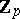-complex.

How to Cite This Entry:
Steenrod reduced power. S.N. MalyginM.M. Postnikov (originator), Encyclopedia of Mathematics. URL: http://encyclopediaofmath.org/index.php?title=Steenrod_reduced_power&oldid=15254
This text originally appeared in Encyclopedia of Mathematics - ISBN 1402006098